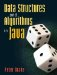# C. Summation Formulae

The algebra of sums is useful when analyzing algorithms (Chapter 7). This appendix reviews some basic summation formulae. Proofs are omitted, but we provide a diagram for each formula to aid in memory.Data Structures and Algorithms in Java
ISBN: 0131469142
EAN: 2147483647
Year: 2004
Pages: 216
Authors: Peter Drake

Similar book on Amazon